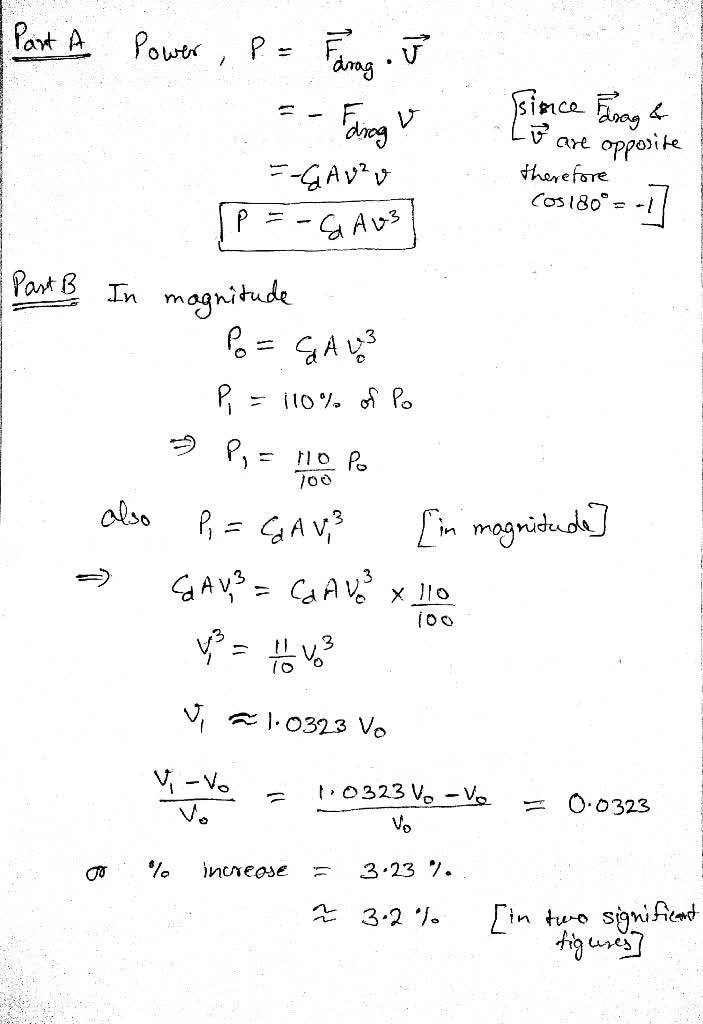The dominant form of drag experienced by vehicles (bikes, cars,planes, etc.) at operating speeds is called form drag. Itincreases quadratically with vsibuzwaW 2021-05-09 Answered
The dominant form of drag experienced by vehicles (bikes, cars,planes, etc.) at operating speeds is called form drag. Itincreases quadratically with velocity (essentially because theamount of air you run into increase with v and so does the amount of force you must exert on each small volume of air). Thus
$$\displaystyle{F}_{{{d}{r}{u}{g}}}={C}_{{d}}{A}{v}^{{2}}$$
where A is the cross-sectional area of the vehicle and $$\displaystyle{C}_{{d}}$$ is called the coefficient of drag.
Part A:
Consider a vehicle moving with constant velocity $$\displaystyle\vec{{{v}}}$$. Find the power dissipated by form drag.
Express your answer in terms of $$\displaystyle{C}_{{d}},{A},$$ and speed v.
Part B:
A certain car has an engine that provides a maximum power $$\displaystyle{P}_{{0}}$$. Suppose that the maximum speed of thee car, $$\displaystyle{v}_{{0}}$$, is limited by a drag force proportional to the square of the speed (as in the previous part). The car engine is now modified, so that the new power $$\displaystyle{P}_{{1}}$$ is 10 percent greater than the original power ($$\displaystyle{P}_{{1}}={110}\%{P}_{{0}}$$).
Assume the following:
The top speed is limited by air drag.
The magnitude of the force of air drag at these speeds is proportional to the square of the speed.
By what percentage, $$\displaystyle{\frac{{{v}_{{1}}-{v}_{{0}}}}{{{v}_{{0}}}}}$$, is the top speed of the car increased?
Express the percent increase in top speed numerically to two significant figures.

• Questions are typically answered in as fast as 30 minutes

Solve your problem for the price of one coffee

• Math expert for every subject
• Pay only if we can solve itRoosevelt Houghton

see my explanation